# How to insert an equation with fractions, square roots and exponents

Word
This tip displays how to add an equation with fractions, square roots and exponents, for example, the normal or Gaussian distribution.

How to add an equation in your document, see Working with Microsoft Equation.

To insert, for example, the normal, or Gaussian distribution, do the following:

## In the Professional format:

1.   In your own equation, enter f(x)=.

2.   Under Equation Tools, on the Design tab, in the Structures group, click the Fraction button: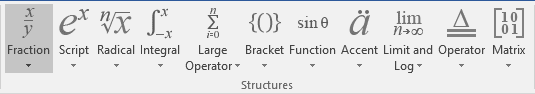In the Fraction list choose Stacked Fraction: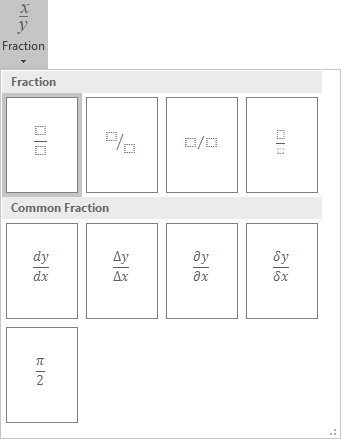3.   Enter 1 at the top of your fraction.

4.   In the bottom of your fraction, do the following:

4.1.   Under Equation Tools, on the Design tab, in the Structures group, click the Radical button. In the Radicals list choose Square root: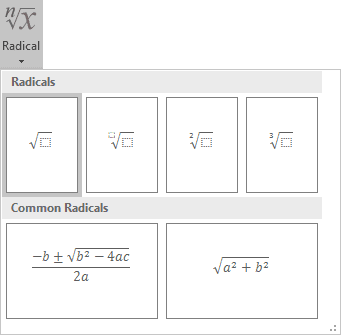4.2.   Enter 2.

4.3.   Under Equation Tools, on the Design tab, in the Symbols group, click the More button: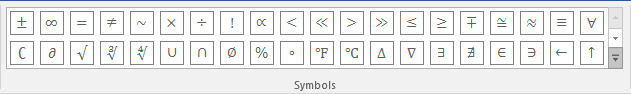In the list of symbols choose: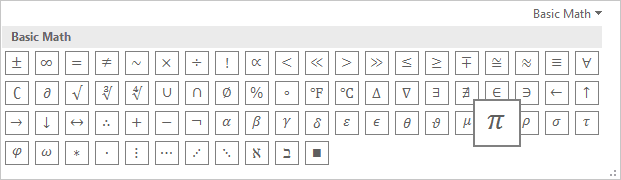4.4.   Under Equation Tools, on the Design tab, in the Structures group, click the Script button. In the Scripts and Superscripts list choose Superscript: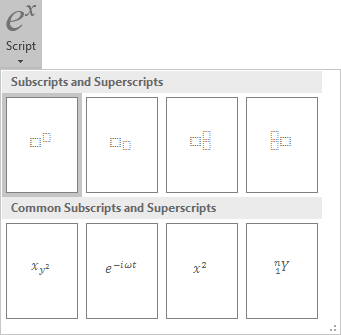4.5.   In the base box of the script, choose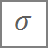.

4.6.   In the upper right box of the script, enter 2.

5.   In the left of your formula choose Script again to enter e in the base box, in the upper right box enter - and choose Fraction again, etc.: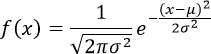## In the Linear format:

1.   In your own equation, enter f(x)=1/.

2.   Under Equation Tools, on the Design tab, in the Symbols group, choose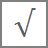or simply \sqrt.

3.   In the brackets enter 2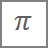(or \pi),(or \sigma) and ^2: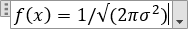Then you enter a space key, this linear formula transformed to the professional format: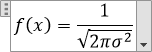4.   Enter e^(-(x-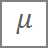(or \mu), )^2/(2,(or \sigma) and then ^2)):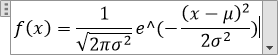Then you enter a space key, the second part of your linear formula transformed to the professional format: